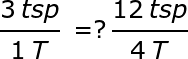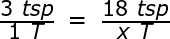# Using Proportions to Solve Ratio Problems

Instructor: Thomas Higginbotham

Tom has taught math / science at secondary & post-secondary, and a K-12 school administrator. He has a B.S. in Biology and a PhD in Curriculum & Instruction.

Proportions allow many different real-world estimations and calculations, and can be done using a straightforward process. In this lesson, learn how to use proportions to solve ratio problems.

## What is a Ratio?

Ratios exist just about anywhere we look. What are they? Simply put, a ratio is two numbers being compared.

For instance, we can compare the number of chocolate chips in a bag to the mass of the bag. The ratio of 250 chips to sixteen ounces can be written in one of three ways.

250 ch: 12 oz.

250 ch to 12 oz.

250 ch / 12 oz.

For the remainder of this lesson, ratios will be written as fractions because it makes calculations easier. However, they could be written in any of the forms shown in the chips example above.

Units are often used in ratios. For example, there are 3 teaspoons (tsp) in every tablespoon (T). This can be written as:

3 tsp / 1 T

Another common value comparison is parts of a whole. For example, in a classroom, there might be 14 girls and 11 boys. The ratio of girls to boys is 14 / 11. We can also write it as the ratio of boys to girls, or 11 / 14. We could compare the number of girls to the total number of students in the class, or 14 / 25. We could compare the number of boys to the total number of students in the class, or 11 / 25.

In each of these examples, we are simply comparing two values - the definition of a ratio.

## Why Would We Care About Ratios?

When ratios are constant, no matter the size or amount you are considering, they can be used to estimate those sizes or amounts for different situations. If we know there are 250 chocolate chips in 12 ounces, we can calculate what mass of chips will give us 3000 chocolate chips. In this example, or in the earlier teaspoons example, we can be very confident that the ratio will be constant, no matter how many chips or teaspoons we are considering.

When we think a ratio might be constant, we can use it to predict. For example, if a basketball player makes 85 of 100 free throws, we can make a prediction about how many free throws that player might make over the course of a week, or a full season of games.

However, if a ratio does not represent a comparison that might hold true over time, it is not predictive. For example, if a professional baseball player has a great day and gets hits on 4 out of 5 at-bats, we can not use that day's ratio of hits to at-bats to predict the number of hits that player will have over a season. Even today's greatest hitters rarely hit for the season at a ratio higher than 3.5 hits per 10 at-bats.

## Predicting and Calculating Using Proportions

A proportion is when two ratios are set equal to each other in an equation. For example, 1 tsp / 3 T = 4 tsp / 12T is a proportion. There are several different ways to know if two ratios are actually equal, but by far the easiest and most general is to cross-multiply, and compare the products. If the products are the same, the ratios are truly proportional. In the example above:3 * 4 = 12

1 * 12 = 12

12 = 12, meaning the ratios are indeed proportional.

Sometimes, however, we have both a ratio with two known values, and a ratio with one known and one unknown value. In that case, we can use the following process to find the unknown value:

1. Identify the ratio with two known values
2. Identify the ratio with one known and one unknown value
3. Set the two ratios in a proportion
4. Cross-multiply
5. Solve for the unknown
Example

A recipe calls for 18 teaspoons of sugar, but you only have a tablespoon with you for measurement. How many tablespoons should you add? Using the steps described above:

1. 3 tsp / 1 T
2. 18 tsp / ? T
3.4. 3x = 18 * 1
5. 3x = 18

x = 6

There are 6 tablespoons in 18 teaspoons. That's some sugar!

## Other Proportion Examples

Example 1

To unlock this lesson you must be a Study.com Member.

### Register to view this lesson

Are you a student or a teacher?